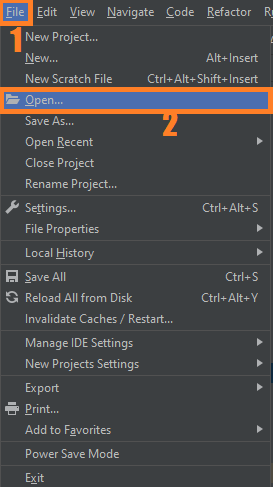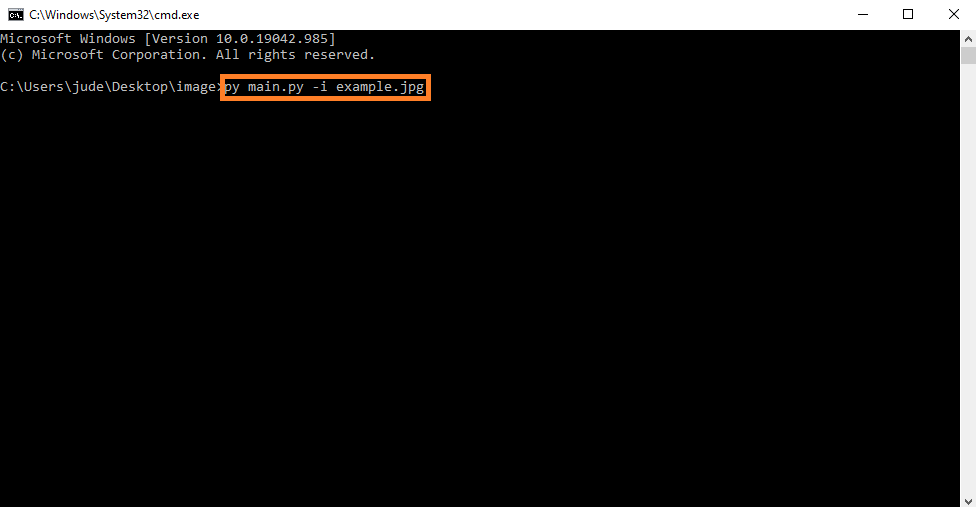# Image Caption Generator with CNN & LSTM In Python With Source Code

## Image Caption Generator with CNN & LSTM In Python With Source Code

The Image Caption Generator with CNN & LSTM In Python was developed using Python Programming with CNN and LSTM. This Project is to learn the concepts of a CNN and LSTM model and build a working model of Image caption generator by implementing CNN with LSTM.

A Image Caption Generator In python we will be implementing the caption generator using CNN (Convolutional Neural Networks) and LSTM (Long short term memory). The image features will be extracted from Xception which is a CNN model trained on the imagenet dataset and then we feed the features into the LSTM model which will be responsible for generating the image captions.

## What is CNN?

Convolutional Neural Networks are specialized deep neural networks which can process the data that has input shape like a 2D matrix. Images are easily represented as a 2D matrix and CNN is very useful in working with images.

CNN is basically used for image classifications and identifying if an image is a bird, a plane or Superman, etc. It scans images from left to right and top to bottom to pull out important features from the image and combines the feature to classify images. It can handle the images that have been translated, rotated, scaled and changes in perspective.

## What is LSTM?

LSTM stands for Long short term memory, they are a type of RNN (recurrent neural network) which is well suited for sequence prediction problems. Based on the previous text, we can predict what the next word will be. It has proven itself effective from the traditional RNN by overcoming the limitations of RNN which had short term memory. LSTM can carry out relevant information throughout the processing of inputs and with a forget gate, it discards non-relevant information.

By the way if you are new to python programming and you don’t know what would be the the Python IDE to use, I have here a list of Best Python IDE for Windows, Linux, Mac OS that will suit for you. I also have here How to Download and Install Latest Version of Python on Windows.

To start executing Image Caption Generator with CNN & LSTM In Python With Source Code, make sure that you have installed Python 3.9 and PyCharm in your computer.

## Image Caption Generator with CNN & LSTM In Python With Source Code : Steps on how to run the project

Time needed: 5 minutes

These are the steps on how to run Image Caption Generator with CNN & LSTM In Python With Source Code

First, download the given source code below and unzip the source code.• Step 2: Import the project to your PyCharm IDE.• Step 3: Run the project.

last, run the project with the command “py main.py -i example.jpg## Installed Libraries

```from keras.preprocessing.text import Tokenizer
from keras.applications.xception import Xception
import numpy as np
from PIL import Image
import matplotlib.pyplot as plt
import argparse```

## Complete Source Code

```from keras.preprocessing.text import Tokenizer
from keras.applications.xception import Xception
import numpy as np
from PIL import Image
import matplotlib.pyplot as plt
import argparse

ap = argparse.ArgumentParser()
args = vars(ap.parse_args())
img_path = args['image']

def extract_features(filename, model):
try:
image = Image.open(filename)

except:
print("ERROR: Couldn't open image! Make sure the image path and extension is correct")
image = image.resize((299,299))
image = np.array(image)
# for images that has 4 channels, we convert them into 3 channels
if image.shape == 4:
image = image[..., :3]
image = np.expand_dims(image, axis=0)
image = image/127.5
image = image - 1.0
feature = model.predict(image)
return feature

def word_for_id(integer, tokenizer):
for word, index in tokenizer.word_index.items():
if index == integer:
return word
return None

def generate_desc(model, tokenizer, photo, max_length):
in_text = 'start'
for i in range(max_length):
sequence = tokenizer.texts_to_sequences([in_text])
pred = model.predict([photo,sequence], verbose=0)
pred = np.argmax(pred)
word = word_for_id(pred, tokenizer)
if word is None:
break
in_text += ' ' + word
if word == 'end':
break
return in_text

#path = 'Flicker8k_Dataset/111537222_07e56d5a30.jpg'
max_length = 32
xception_model = Xception(include_top=False, pooling="avg")

photo = extract_features(img_path, xception_model)
img = Image.open(img_path)

description = generate_desc(model, tokenizer, photo, max_length)
print("\n\n")
print(description)
plt.imshow(img)

```